Have a lot of worksheets but the worksheet names are arranged in a random order? It will be a pain moving the sheets one by one to sort them out! You can sort all worksheets by name using Macros in Excel!

Make sure your Excel has the Developer Tab enabled following this tutorial.

I explain how you can do this below step by step!

What does it do?

Sort all worksheets by name alphabetically

Copy Source Code:

```Sub SortAllWorksheetsByName()

Dim i As Integer
Dim j As Integer

'We use two loops to sort the sheets in ascending order
For i = 1 To Sheets.Count
For j = 1 To Sheets.Count - 1
If UCase\$(Sheets(j).Name) > UCase\$(Sheets(j + 1).Name) Then
Sheets(j).Move After:=Sheets(j + 1)
End If
Next j
Next i

End Sub

```

Final Result: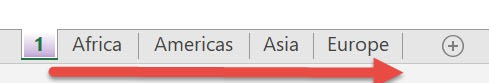Exercise Workbook:

This is our worksheet ordering: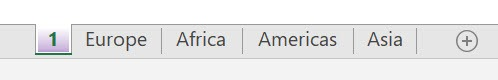STEP 1: Go to Developer > Code > Visual Basic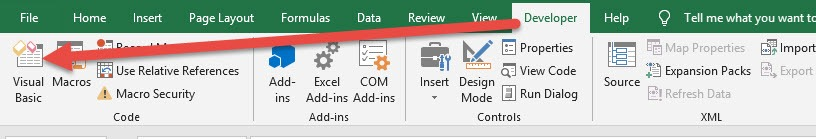STEP 2: Paste in your code and Select Save. Close the window afterwards.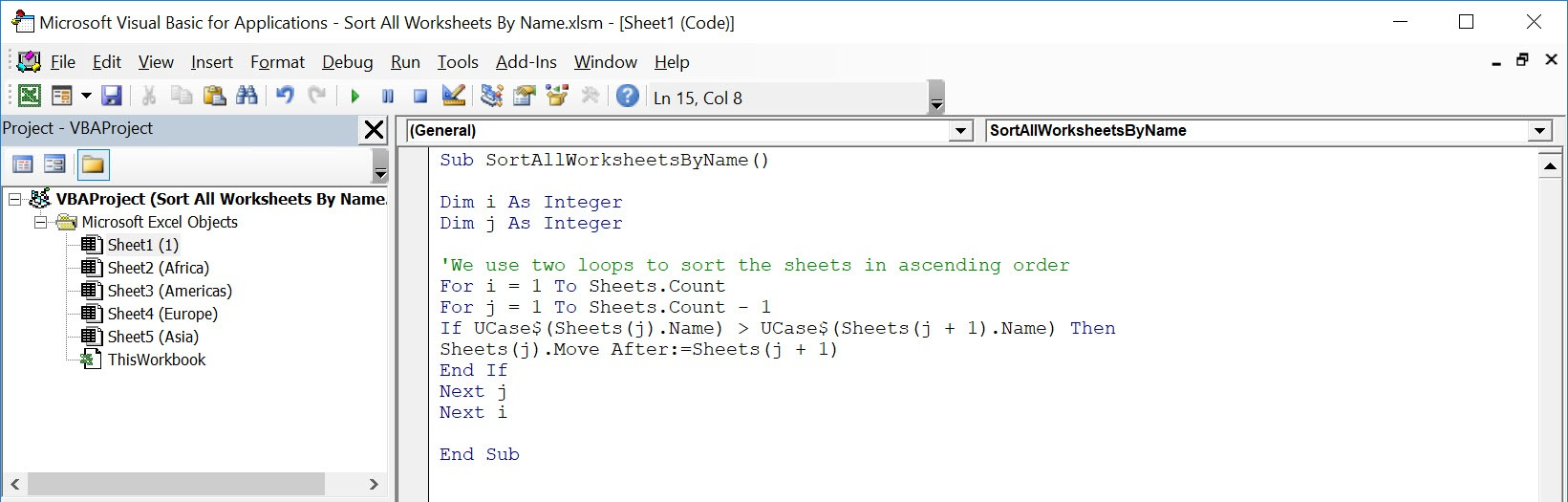STEP 3: Let us test it out!

Open the sheet containing the data. Go to Developer > Code > Macros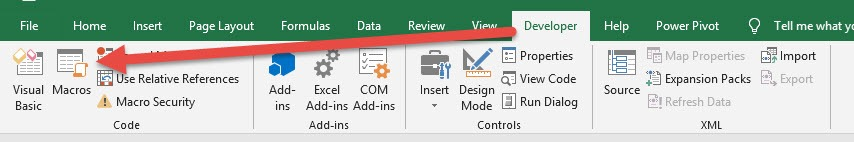Make sure your macro is selected. Click Run.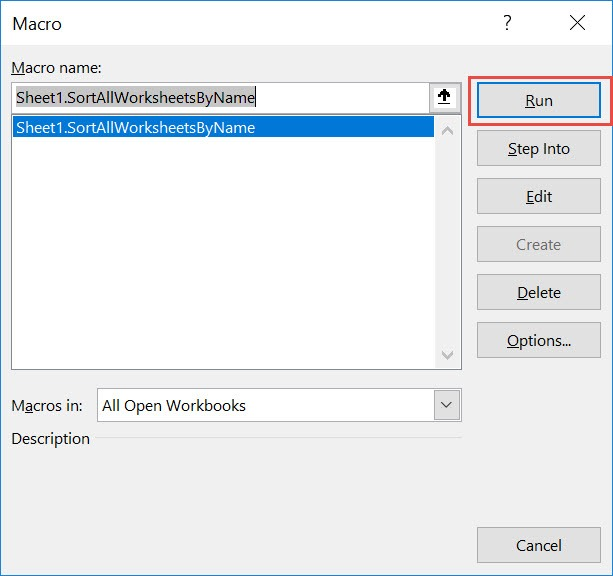With just one click, all of the worksheets are now sorted alphabetically!How to Sort All Worksheets By Name Using Macros in Excel